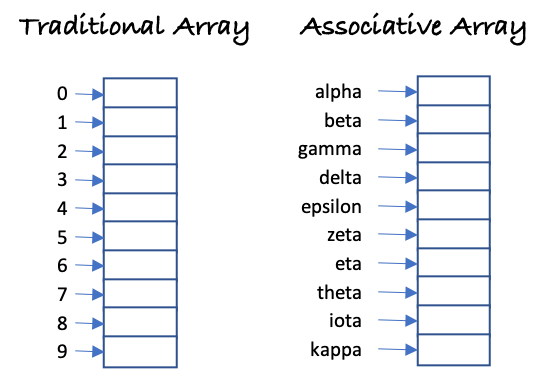This is another article in the group of data structures.

A hash map is a powerful in-memory data structure that allows adding and retrieving data in a constant time. Most modern languages have support for this and usually they include variations and optimizations over it, therefore most of us take this for granted, but this was not always the case, and here I try to explain how this one works. The basic data structure supported by any programming language is the array. An array is a contiguous block of memory set aside to store the same type of data. For example an array of 10 integers, will occupy in a 64 bit machine 80 bytes. An array is quite powerful because you can write and retrieve values from and to the array by using a single operation, which is denoted as O(1) using the Big O notation.

What a hash map solves is a problem where we want to have a set of data indexed instead of by numbers, by an arbitrary type of data, this is known as an associative array because each value in the array is associated with a key instead of a numeric index assigned by the value’s position within the array. But of course the order of the data within the associative array is not given by any position. Conceptually it can be visualized as the following image:In the left hand side we have a basic array, indexed by the position in memory (and this is the reason why an array starts with index 0), while on the right hand side we have an associative array indexed by an arbitrary key. If we stored any value in the element associated with “alpha” we would be overwriting the element there.

The way to achieve this, first is to create an array with a fixed size that is capable of holding as many elements as we need, and then using a hash function that translates the key into a unique integer value. Once we have an integer value, we can simply calculate the hash key modulo the size of the array to find the location of the element in the array.

Having a unique key and having the same key every time each element requires computing it is a must for the above description to work. What happens after that with the key can change depending on the implementation. Having a large integer module a shorter one (the size of the array) will lead inevitably to what is called collisions. That means two or more keys will end up pointing to the same element in the array. To solve the collision, one way is to generate a single linked list that starts in the element of the array indicated by the hash key. Another option is to start calculating the (key + i) modulo (array size). Here i will mean how many iterations have to happen for us to find an empty spot in the table. In my opinion the first one is a cleaner one and the one I have used for years.

If we wanted to calculate a simple hash value for the string in the input box below, we can use the following code

Enter text: Hash:

Javascript already provides associative arrays built, therefore there is no need to create a hash map, however for example purposes we can implement a simple one following the guidelines above with the following code, instead of writing pseudocode I prefer giving fully working javascript that can be run in this same browser or adapted to your preferred language.

Please let me know below your comments, and what other programming challenges you would like to get explained here.